# TensorFlow2.1入门学习笔记(3)——Pillow数字图像处理

## TensorFlow2.0学习笔记(3)——Pillow数字图像处理基础

##### 1.数字图像的基本概念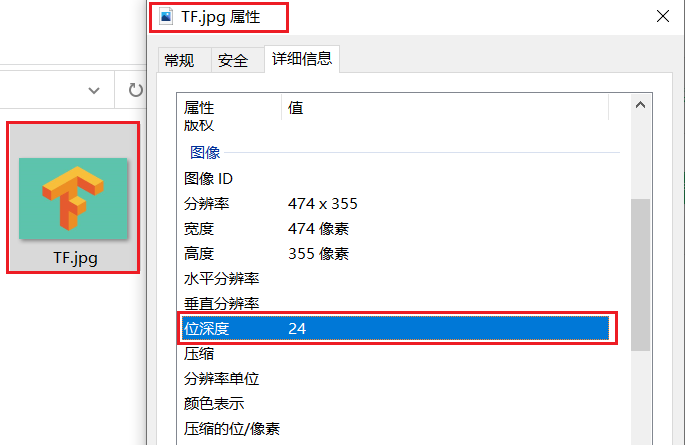EMP：Windows系统的标准位图格式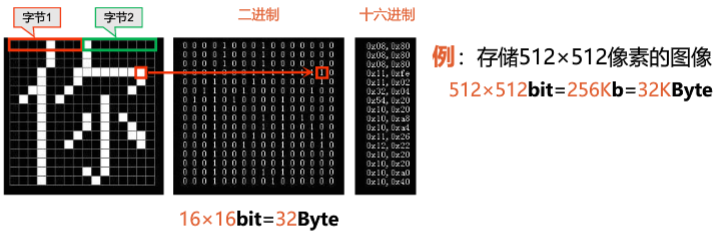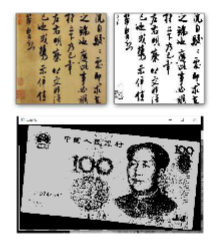PS：只要仅有两种颜色的图像，都可以被称为二值图像，区分于灰度图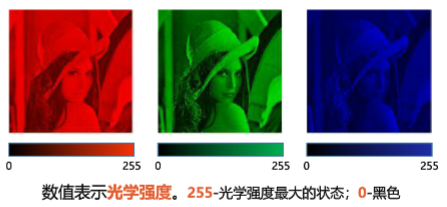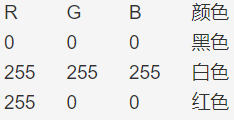RGB为24位真彩色RGBA图像——32位真彩色
RGB图像+8位透明度信息Alpha，1一个像素使用4个字节，位深度为32位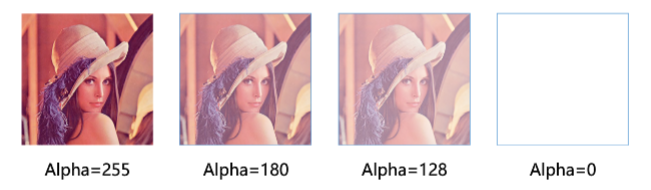256色彩色图像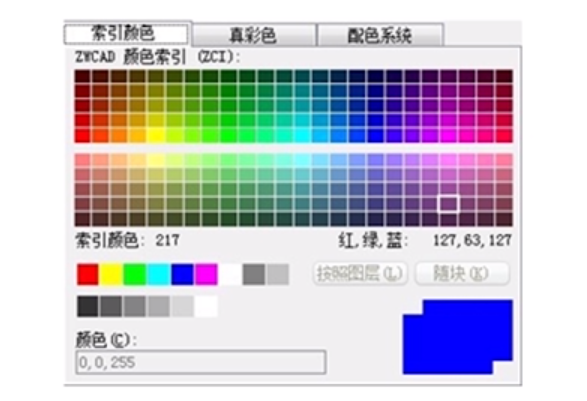BMP格式：占用空间大，不支持文件压缩，不适用于网页
JPEG格式：有损压缩，压缩效率高，所占空间小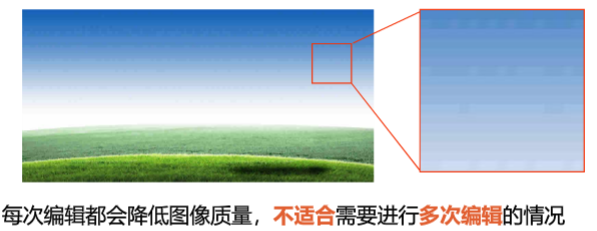PNG格式：无损压缩；适合有规律的渐变色彩的图像，广泛运用于网络GIF格式：支持静态格式和动态格式；动态图片由多幅图片保存为一个图片，循环显示，形成动画效果；只支持256色，适用于色彩简单。颜色较少的小图像

CMYK——印刷四分色：C（cyan=青色）、M（magenta=洋红色）、Y（yellow=黄色）、K（black=黑色）
YCbCr——Y（亮度）、Cb（蓝色色度）、Cr（红色色度）
HSI——H（色调）、S（饱和度）、I（亮度）##### 2.Pillow图像处理库

Pillow的安装

pip install -i https://pypi.tuna.tsinghua.edu.cn/simple pillow


Pillow.image的导入

from PIL import Image

###### 常用函数

Image.open()函数：打开图像
Image.open(路径)：返回值为image对象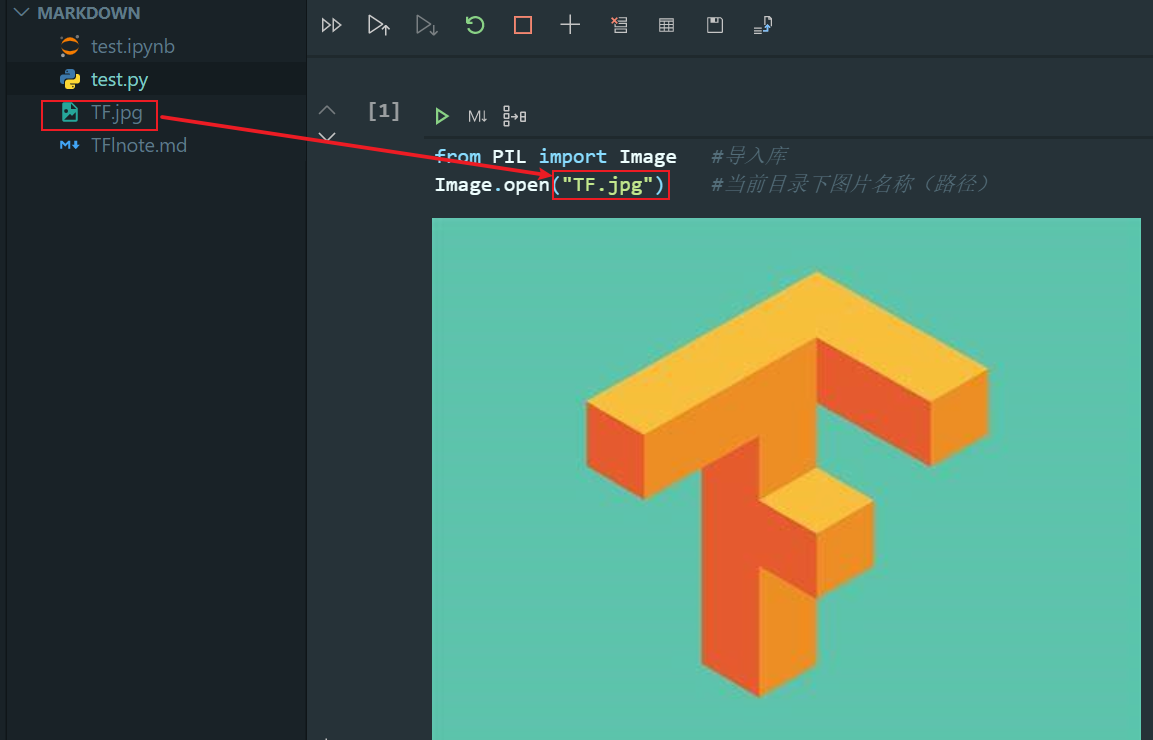Image.save()函数：保存图像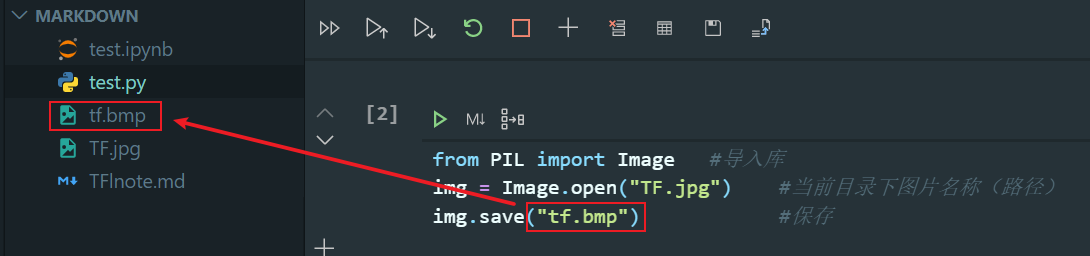from PIL import Image   #导入库
img = Image.open("TF.jpg")    #当前目录下图片名称（路径）
print(img.format)       #JPEG 图像格式
print(img.size)         #(473, 349) 图像尺寸
print(img.mode)         #RGB 色彩模式


imshow()显示图像

plt.imshow(image对象/Numpy数组)

from PIL import Image
import matplotlib.pyplot as plt
img = Image.open("TF.jpg")
plt.figure(figsize=(5,5))      #创建画布
plt.imshow(img)                #画图
plt.title(img.format)          #在标题显示图片格式
plt.show()                     #显示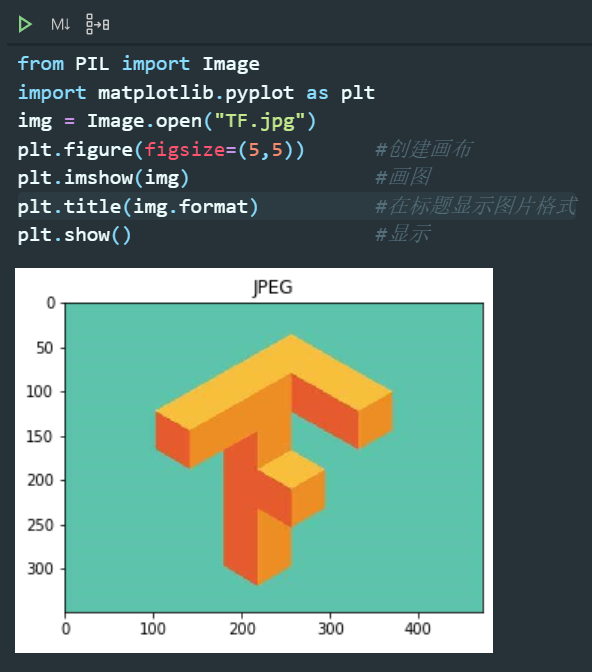convert()函数——转换图像的色彩模式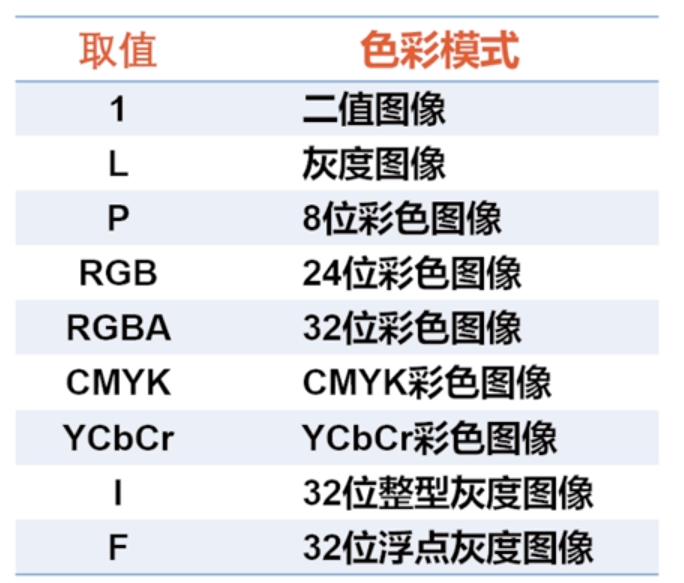img_gray=img.convert("L")
print(img_gray.mode)
plt.figure(figsize=(5,5))
plt.imshow(img)
plt.show()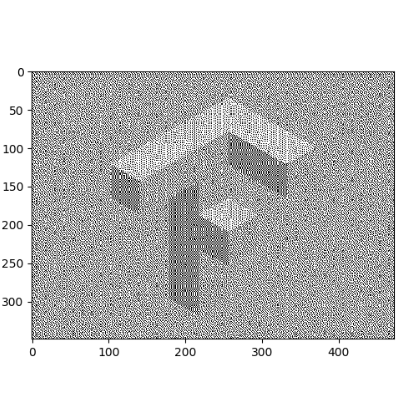from PIL import Image
import matplotlib.pyplot as plt #导入库
img = Image.open("TF.jpg")      #打开文件
img_r,img_g,img_b = img.split() #通道分离
plt.figure(figsize=(10,10))     #创建画布

plt.subplot(2,2,1)              #创建2x2的子图
plt.axis("off")                 #不显示坐标轴
plt.imshow(img_r,cmap="gray")   #以灰度图显示r通道
plt.title("R",fontsize=20)      #创建子图标题

plt.subplot(2,2,2)
plt.axis("off")
plt.imshow(img_g,cmap="gray")
plt.title("G",fontsize=20)

plt.subplot(2,2,3)
plt.axis("off")
plt.imshow(img_b,cmap="gray")
plt.title("B",fontsize=20)

plt.subplot(2,2,4)
img_rgb=Image.merge("RGB",[img_r,img_g,img_b])  #通道合并
plt.axis("off")
plt.imshow(img_rgb)
plt.title("RGB",fontsize=20)
plt.show()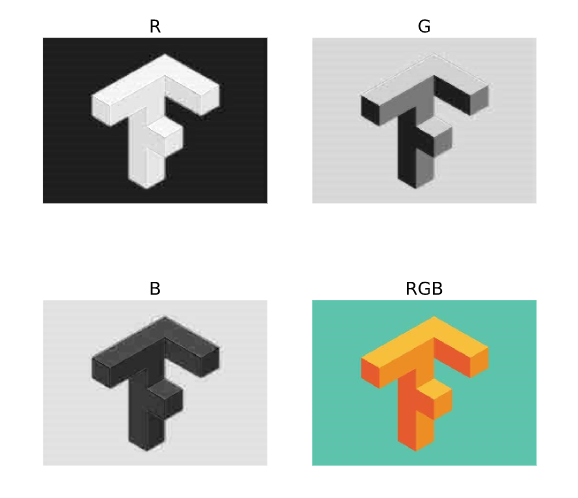np.array(图像对象)

from PIL import Image
import numpy as np
import matplotlib.pyplot as plt
img = Image.open("TF.jpg")
arr_img=np.array(img)
print(arr_img.shape)			#(349, 473, 3)
print(arr_img)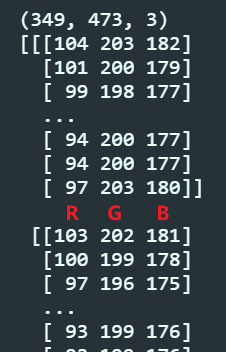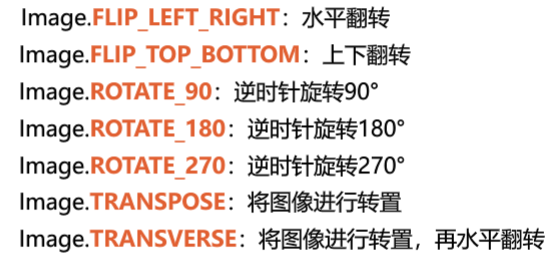from PIL import Image
import numpy as np
import matplotlib.pyplot as plt #导入库

plt.rcParams['font.sans-serif']="SimHei"
img = Image.open("TF.jpg")      #打开文件

plt.figure(figsize=(10,10))     #创建画布

plt.subplot(3,2,1)
plt.axis("off")
plt.imshow(img)
plt.title("原图",fontsize=20)

plt.subplot(3,2,2)
plt.axis("off")
img_arr=np.array(img)
img_arr_new=255-img_arr             #颜色反向处理
plt.imshow(img_arr_new)
plt.title("颜色反向",fontsize=20)

plt.subplot(3,2,3)
plt.axis("off")
plt.imshow(img)
plt.title("原图",fontsize=20)

plt.subplot(3,2,4)
plt.axis("off")
img_flr=img.transpose(Image.FLIP_LEFT_RIGHT)
plt.imshow(img_flr)
plt.title("左右翻转",fontsize=20)

plt.subplot(3,2,5)
plt.axis("off")
img_r90=img.transpose(Image.ROTATE_90)
plt.imshow(img_r90)
plt.title("逆时针旋转90度",fontsize=20)

plt.subplot(3,2,6)
plt.axis("off")
img_tp=img.transpose(Image.TRANSPOSE)
plt.imshow(img_tp)
plt.title("转置",fontsize=20)

plt.show()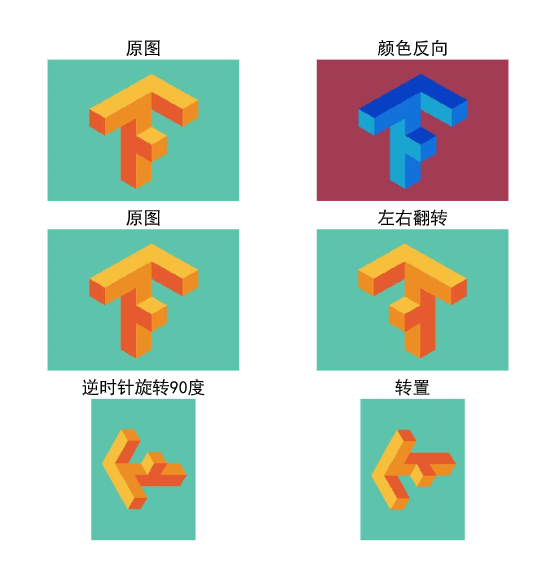from PIL import Image
import matplotlib.pyplot as plt
img = Image.open("TF.jpg")
plt.figure(figsize=(10,10))
plt.subplot(1,2,1)
plt.imshow(img)

plt.subplot(1,2,2)
img_region=img.crop((100,100,300,300))
plt.imshow(img_region)

plt.show()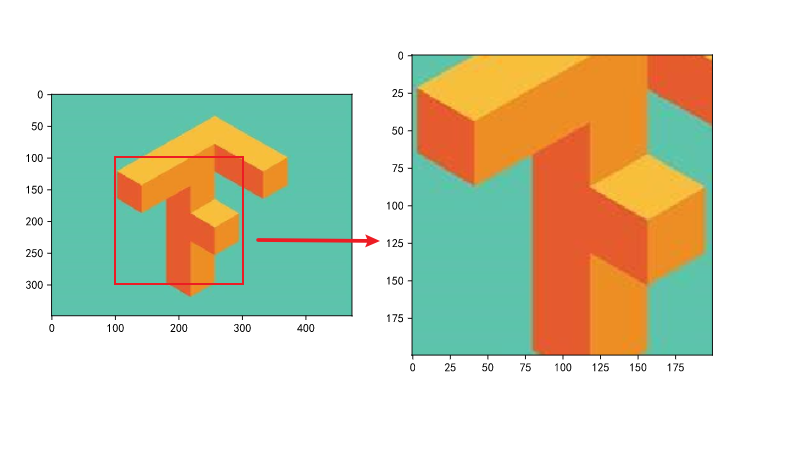SUMMARIZE: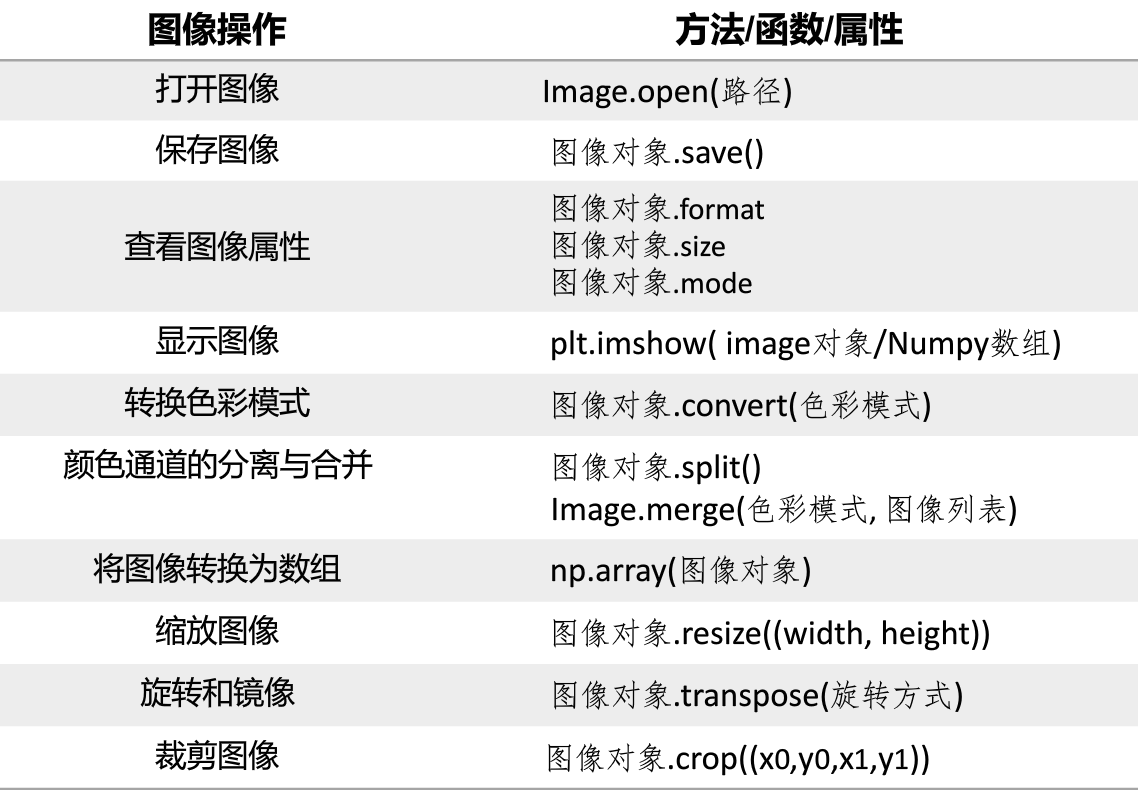CSDN链接

posted @ 2020-05-07 10:57  Moonx5  阅读(659)  评论(0编辑  收藏  举报